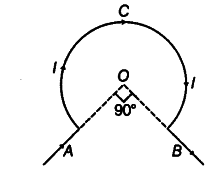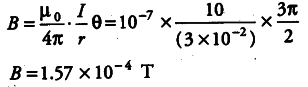# The wire shown in the figure, carries a current of 10 A

The wire shown in the figure, carries a current of 10 A. Determine the magnitude of magnetic field induction at the centre O. Given the radius of bent coil is 3 cm.Here, / = 10 A, r = 3 cm, r = 3 X {{10}^{-2}}
Angle subtended by coil at the centre,
θ = 360° - 90° = 270 ° = 3$\pi$ / 2 rad
Magnetic field induction at O due to current through circular path ACB is,